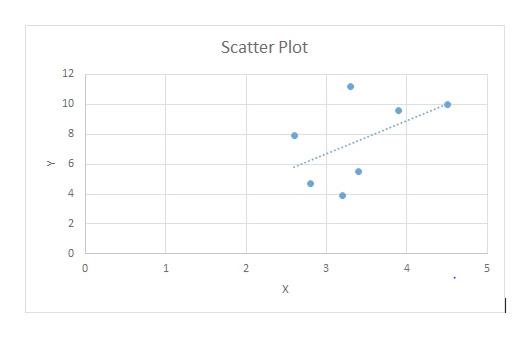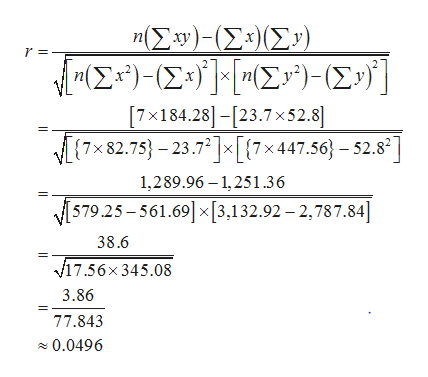Is the magnitude of an earthquake related to the depth below the surface at which the quake occurs? Let x be the magnitude of an earthquake (on the Richter scale), and let y be the depth (in kilometers) of the quake below the surface at the epicenter.x2.83.93.34.52.63.23.4y4.79.611.210.07.93.95.5(a) Make a scatter diagram of the data. Flash Player version 10 or higher is required for this question. You can get Flash Player free from Adobe's website. Then visualize the line you think best fits the data.(b) Use a calculator to verify that Σx = 23.7, Σx2 = 82.75, Σy = 52.8, Σy2 = 447.56 and Σxy = 184.28.Compute r. (Round to 3 decimal places.) As x increases, does the value of r imply that y should tend to increase or decrease? Explain your answer.Given our value of r, we can not draw any conclusions for the behavior of y as x increases.Given our value of r, y should tend to remain constant as x increases.   Given our value of r, y should tend to decrease as x increases.Given our value of r, y should tend to increase as x increases.

Question

Is the magnitude of an earthquake related to the depth below the surface at which the quake occurs? Let x be the magnitude of an earthquake (on the Richter scale), and let y be the depth (in kilometers) of the quake below the surface at the epicenter.

 x 2.8 3.9 3.3 4.5 2.6 3.2 3.4 y 4.7 9.6 11.2 10 7.9 3.9 5.5
(a) Make a scatter diagram of the data.

Flash Player version 10 or higher is required for this question.
You can get Flash Player free from Adobe's website.

Then visualize the line you think best fits the data.

(b) Use a calculator to verify that Σx = 23.7, Σx2 = 82.75, Σy = 52.8, Σy2 = 447.56 and Σxy = 184.28.

Compute r. (Round to 3 decimal places.)

As x increases, does the value of r imply that y should tend to increase or decrease? Explain your answer.
Given our value of r, we can not draw any conclusions for the behavior of y as x increases.
Given our value of ry should tend to remain constant as x increases.
Given our value of ry should tend to decrease as x increases.
Given our value of ry should tend to increase as x increases.
Step 1

Part (a):

The scatter diagram, along with the best fitted line (least-squares regression line) for the data is as follows:help_outlineImage TranscriptioncloseScatter Plot 12 10 6 4 2 2 1 0 X st fullscreen
Step 2

Part (b):

Here, ∑x, ∑x2, ∑y, ∑y2, and ∑xy are the sums of X, X2 Y, Y2, and XY, respectively.

Using calculator, it is verified that:

x = (2.8 + 3.9 + 3.3 + 4.5 + 2.6 + 3.2 + 3.4) = 23.7.

x2 = (2.82 + 3.92 + 3.32 + 4.52 + 2.62+3.22 +3.42) = 82.75.

y = (4.7 + 9.6 + 11.2 + 10.0 + 7.9 + 3.9+ 5.5) = 52.8.

y2 = (4.72 + 9.62 + 11.22 + 102 + 7.92 +3.92 +5.52) = 447.56.

xy = (2.8×4.7) + (3.9×9.6) + (3.3×11.2) + (4.5×10) + (2.6×7.9) + (3.2×3.9) + (3.4×5.5) = 184.28.

There are n = 7 pairs of observations.

Using the given notations, the correlation coefficient is calculated below:help_outlineImage TranscriptioncloseΣw)- Σ:) (Σ) [ΙΣ)- (Σ-) ΣΥ)- (Σy} | η [7x184.28]-[23.7x52.8] 7x82.75 -23.72x{7x447.56}- 52.82 1,289.96 1,251.36 579.25-561.69 [3,132.92 -2,787.84] 38.6 v17.56x345.08 3.86 77.843 0.0496 fullscreen
Step 3

Interpretation:

The correlation coefficient is positive. This means that, as x increases from 2.8 to 3.4, given our valu...

Want to see the full answer?

See Solution

Want to see this answer and more?

Our solutions are written by experts, many with advanced degrees, and available 24/7

See Solution
Tagged in

Other